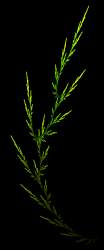News: Visit us on facebook## The All New FractalForums is now in Public Beta Testing! Visit FractalForums.org and check it out!

GreensPrevious Image | Next Image
Description: First time I got anything resembling volumetric light (coincidence!), and also first time I had the idea I had a tiny bit of control about the colours :} YAYAmazing Surface by Kali, tweak of 'Has issues' by 3dickulus, original timemit and Crist-JRoger

Code:
#info Amazing Surface by Kali - Based on Tglad's Amazing Box

#define providesInit
#define USE_INF_NORM
#define KN_VOLUMETRIC

#include "MathUtils.frag"

#include "DE-Kn2cr11.frag"

#group AmazingSurface
uniform int Iterations;  slider[0,17,300]
uniform int ColorIterations;  slider[0,3,300]
uniform float Scale;  slider[-3,1.5,3.0]
uniform int FoldType; slider[1,1,3]
uniform vec3 PreTranslation; slider[(-5,-5,-5),(0,0,0),(5,5,5)]
uniform vec2 FoldValues; slider[(0,0),(1,1),(5,5)]
uniform bool Julia; checkbox[false]
uniform vec3 JuliaValues; slider[(-5,-5,-5),(-1,-1,-1),(5,5,5)]
uniform vec3 RotVector; slider[(-1,-1,-1),(1,1,1),(1,1,1)]
uniform float RotAngle; slider[-180,0,180]

mat3 rot;

void init() {
rot = rotationMatrix3(normalize(RotVector), RotAngle);
}

vec4 scale = vec4(Scale, Scale, Scale, abs(Scale))/MinRad2;
float absScalem1 = abs(1-Scale);
float AbsScaleRaisedTo1mIters = pow(abs(Scale), float(1-Iterations));

float DE(vec3 pos) {
vec4 p = vec4(pos,1);
vec3 c = Julia?JuliaValues:p.xyz;
for (int i=0; i<Iterations; i++) {
if (FoldType==1) p.xy=abs(p.xy+FoldValues)-abs(p.xy-FoldValues)-p.xy;
if (FoldType==2) p.xy=FoldValues-abs(abs(p.xy)-FoldValues);
if (FoldType==3) p.xy=abs(p.xy+FoldValues);
p.xyz+=PreTranslation;
float r2 = dot(p.xyz, p.xyz);
if (i<ColorIterations) orbitTrap = min(orbitTrap, abs(vec4(p.xyz,r2)));
p = p*scale;
p.xyz+=c;
p.xyz*=rot;
}
return ((length(p.xyz) - absScalem1) / p.w - AbsScaleRaisedTo1mIters);
}

#preset default
FOV = 0.6101695
Eye = 8.949551,4.595715,1.071182
Target = 5.338451,2.198186,3.524075
UpLock = false
Up = -0.1758244,-0.1016364,-0.3581875
FocalPlane = 1.79281
Aperture = 0.0699153
InFocusAWidth = 0.97354
DofCorrect = false
ApertureNbrSides = 5
ApertureRot = 186.707
ApStarShaped = false
Gamma = 1.4
ToneMapping = 5
Exposure = 1.5
Brightness = 1
Contrast = 0.7083043
Saturation = 1
GaussianWeight = 0.798838
AntiAliasScale = 0.3669725
Bloom = false
BloomIntensity = 2
BloomPow = 2
BloomTaps = 30
BloomStrong = 1
Detail = -3.617647
RefineSteps = 9
FudgeFactor = 0.75
MaxRaySteps = 512
MaxDistance = 4.5
Dither = 1
NormalBackStep = 1
DetailAO = -2.751379
coneApertureAO = 1
maxIterAO = 40
FudgeAO = 1
AO_ambient = 1
AO_camlight = 1.5
AO_pointlight = 1
AoCorrect = 0
Specular = 0.0533333
SpecularExp = 5
CamLight = 0.756863,0.937255,0.937255,0
AmbiantLight = 0.913725,0.968627,1,1
Reflection = 0.207843,0.184314,0.2
ReflectionsNumber = 0
SpotGlow = true
SpotLight = 0.823529,1,1,10
LightPos = 4.251242,6.664301,1.738822
LightSize = 0.19345
LightFallOff = 0.77222
LightGlowExp = 1.83545
perf = false
SSS = false
sss1 = 0.1
sss2 = 0.5
BaseColor = 0.6,0.619608,0.65098
OrbitStrength = 1
X = 0.1607843,0.2078431,0.2078431,1
Y = 0.9176471,0.07843137,0.01960784,1
Z = 0.9764706,0.6117647,0.1647059,1
R = 0.05490196,0.8745098,0.03921569,0.2094972
BackgroundColor = 0.137255,0.337255,0.576471
CycleColors = false
Cycles = 25.58234
EnableFloor = false
FloorNormal = 0,0,1
FloorHeight = 0.5053
FloorColor = 1,1,1
HF_Fallof = 0.0005
HF_Const = 0
HF_Intensity = 0.2073602
HF_Dir = 0.58648,-0.3011,-0.39662
HF_Offset = -10
HF_Color = 0.835294,0.956863,0.984314,0.1265189
HF_Scatter = 1
HF_Anisotropy = 0,0,0
HF_FogIter = 1
EnCloudsDir = true NotLocked
Clouds_Dir = 0,0,1 NotLocked
CloudScale = 0.24602 NotLocked
CloudFlatness = 0.08167 NotLocked
CloudTops = -10 NotLocked
CloudBase = -10 NotLocked
CloudDensity = 0.13491 NotLocked
CloudRoughness = 1.56126 NotLocked
CloudContrast = 5.9297 NotLocked
CloudColor = 0.905882,0.886275,0.588235 NotLocked
CloudColor2 = 0.07,0.17,0.24 NotLocked
SunLightColor = 0.698039,0.203922,0.105882 NotLocked
Cloudvar1 = 0.99 NotLocked
Cloudvar2 = 1 NotLocked
CloudIter = 5 NotLocked
CloudBgMix = 1 NotLocked
Iterations = 56
ColorIterations = 20
Scale = -1.273234
FoldType = 3
PreTranslation = -3.174603,-1.053391,-3.968254
FoldValues = 3.029119,5
Julia = true
JuliaValues = -0.907781,0.965418,-1.54899
RotVector = 0.400862,0.29454,0.54023
RotAngle = -24.09449
#endpreset

Stats:
Total Favorities: 0 View Who Favorited
Filesize: 3.84MB
Height: 1080 Width: 1920
Discussion Topic: View Topic
Keywords: Fragmentarium
Posted by: SabineSeptember 21, 2016, 07:16:22 PM

Rating: Has not been rated yet.

 Ever GreensRating: (None)Filesize: 720.05kBDate: June 08, 2010, 03:48:09 PMComments (0)By: Bent-Winged Angel